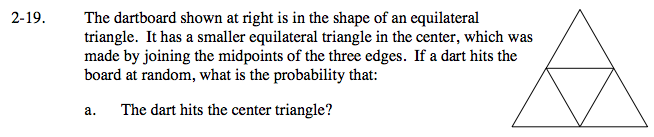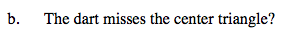### Home > AC > Chapter 14 > Lesson 14.2.1.1 > Problem2-19

2-19.How many smaller triangles make up the bigger triangle?

$\text{The probability is }\frac{1}{4}.$How many smaller triangles can be hit if the center one is missed?